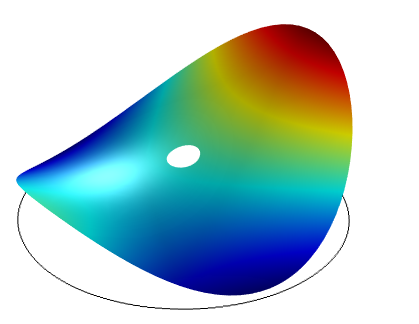# 利用 COMSOL Multiphysics 拟合实验数据曲线

2015年 3月 19日

### 作为最小化问题的曲线拟合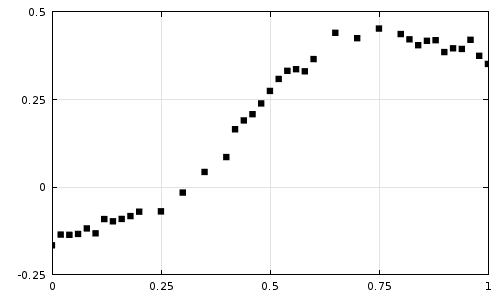\int_a^b (D(x)- F(x))^2 dx

F(x) = c_0\left(\frac{b-x}{b-a}\right)^3 + c_1 \left(\frac{x-a}{b-a}\right)\left(\frac{b-x}{b-a}\right)^2 + c_2 \left(\frac{x-a}{b-a}\right)^2\left(\frac{b-x}{b-a}\right) + c_3 \left(\frac{x-a}{b-a}\right)^3

F(x) = c_0(1-x)^3 + c_1 x(1-x)^2 + c_2 x^2(1-x) + c_3 x^3

R(c_0,c_1,c_2,c_3,x)= \int_a^b (D(x)- F(c_0,c_1,c_2,c_3,x))^2 dx

\frac{\partial R} {\partial c_0} = \frac{\partial R} {\partial c_1} = \frac{\partial R} {\partial c_2} =\frac{\partial R} {\partial c_3} = 0

### 在 COMSOL 中执行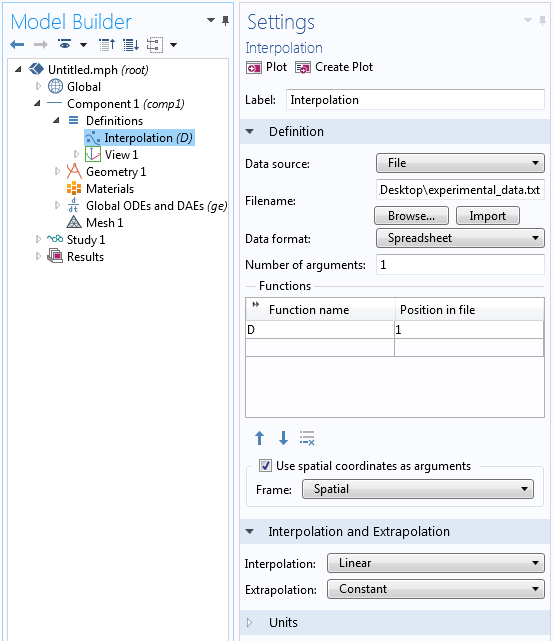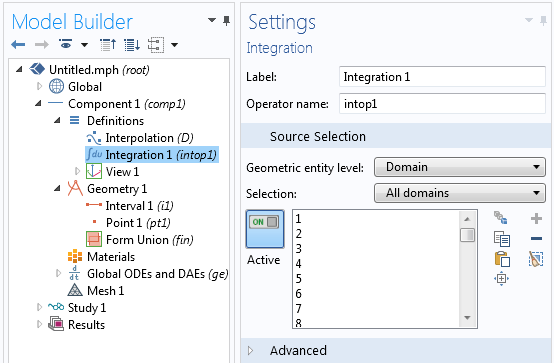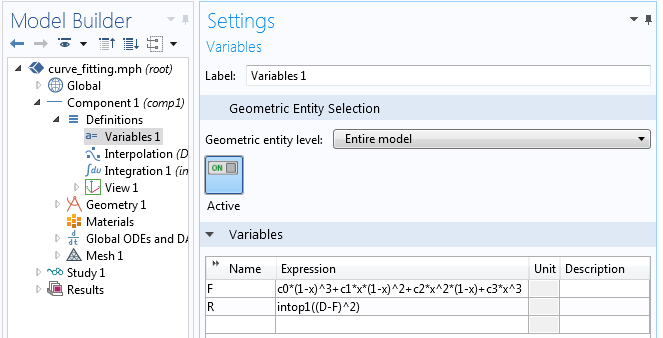‘全局方程’用于求解拟合函数的系数。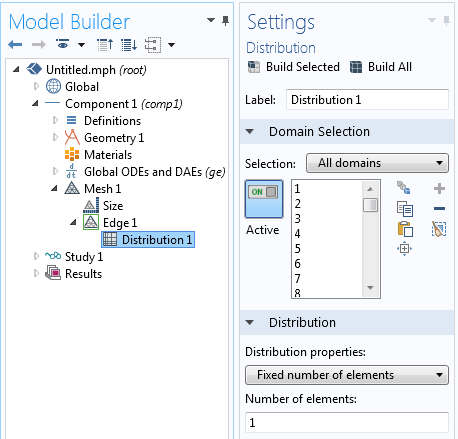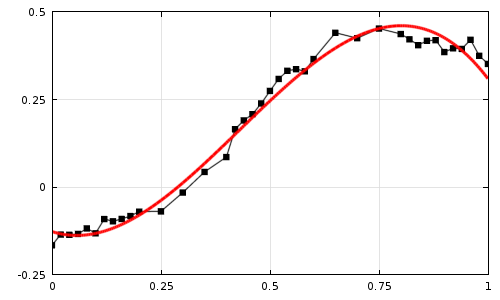### 进一步拓展

F(x) = c_0 + c_1sin ( \pi x /4 ) + c_2cos ( \pi x /4 ) + c_3sin ( \pi x /2 ) + c_4cos ( \pi x /2 )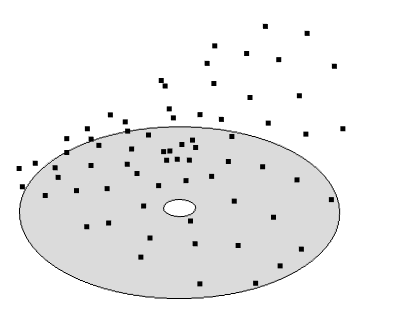F(x) = c_0 + c_1r cos ( \theta ) +c_2 r sin ( \theta )+ c_3(2r^2-1) + c_4 r^2 cos ( 2\theta ) +c_5 r^2 sin ( 2\theta )